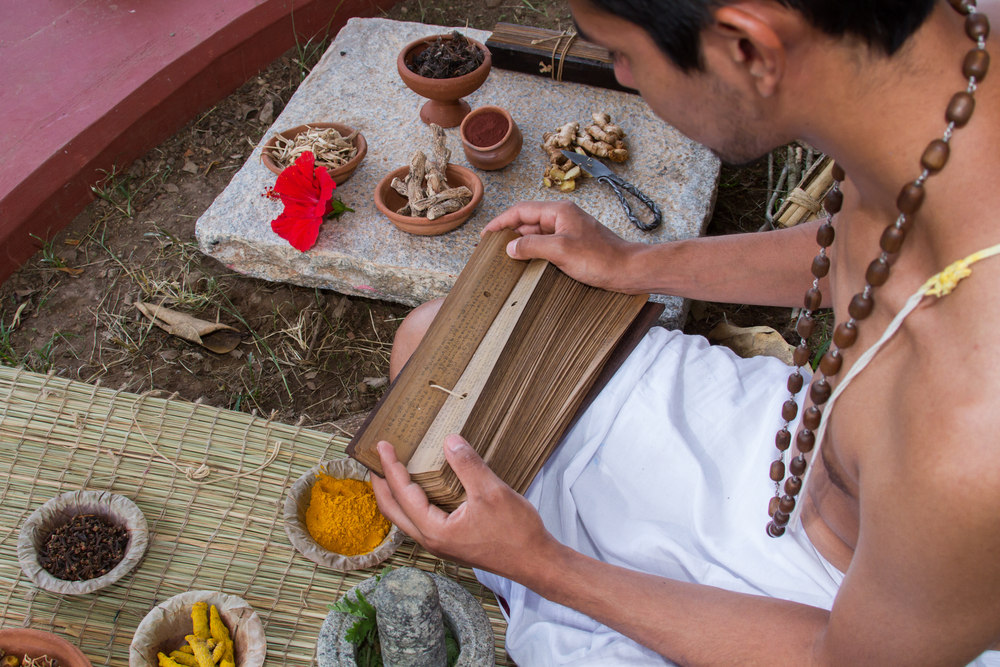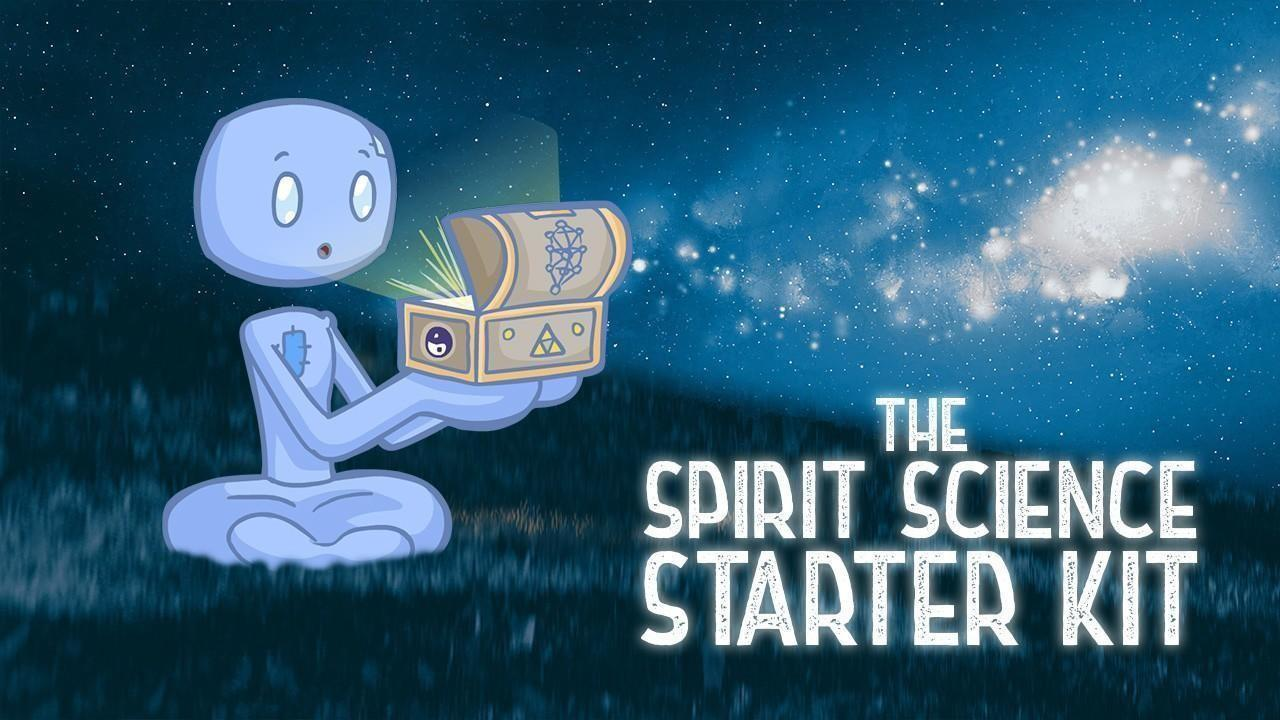# Vedic Math: A Different Way of Thinking

Mar 09, 2020Do math problems scare and intimidate you? Have you ever had math thoughts and just felt so frustrated because you found it so confusing? There’s a way to make math easier and much more fun. It is through Vedic Math. It is a system of math techniques for doing math problems. This system is based on Veda, which is an ancient Indian teaching.

## What is Vedic Math?

In Vedic Math classes, mathematics is not hard to learn and use. The technique can simplify arithmetic and even algebraic operations. Many people around the world find it useful. If you’re having trouble with math in your daily life, you may benefit from learning the rules of Vedic Math and Vedic math formulas.

Many experts state that Vedic Math is a handy tool for students or even individuals who need to solve math problems fast. If you want to be prepared for exams that require mathematical computations or just need to improve this skill, then Vedic Math can help.## History of Vedic Math

Vedic Math is called such because it comes from Vedas. In particular, this technique comes from Atharva Vedas, which is the fourth Veda. Atharva Veda focuses on dealing with the fields of medicine, sculpture, engineering, and mathematics, to name a few. As you can see, Vedic Math can be applied to almost all areas of science.

Veda is a Sanskrit word that comes from the root word Vid which means to learn without limitations. It is the repository of all human knowledge and is fathomless. It is always revealing something as you delve on it deeper.

With Vedic Math, you can apply it to arithmetics, number theory, compound multiplications, factorizations, algebraic operations, simple quadratic as well as higher-order equations, partial fractions, simultaneous quadratic equations, calculus, cubing, squaring, cube root, square root, as well as coordinate geometry and many others.

## Benefits of Learning Vedic Math TechniquesThere are many benefits to learning and using Vedic Math. Here are some of them:

• You will be able to solve math problems much faster.
• As a student, it reduces a lot of the burden of learning more complex operations.
• It reduces the need to finger count and do scratch work.
• Your concentration will improve as you practice more.
• It can reduce avoidable mistakes when solving math problems.

Vedic Mathematics is what Sri Bharati Krsna Tirthaji called this technique. It is from the ancient Indian Mathematics system, which he rediscovered in the early 20th Century. In his research, all maths are based on the 16 Sutras or word-formulas. An example here is “Vertically and Crosswise,” which is one of the 16 Sutras. The word-formulas can describe the manner in which the mind works naturally. In doing so, it’s a huge help in teaching and directing students on the right methods of solving mathematical problems.

The Vedic Math system is coherent which makes it really interesting. It is not merely a combination of unrelated systems. Instead, they are intricately interrelated as well as unified. In multiplication, for example, you can do one-line divisions if you reverse it. For the squaring method, you can reverse it so you can derive one-line square roots. It makes math so much easier and more fun to do. Vedic Math also encourages students to be innovative in their techniques.

## Where can it be Applied?

Vedic Math is not limited to solving simple problems. It can also tackle more difficult problems. You’ll find that when you apply Vedic Math, you can solve problems immediately. Such beautiful methods are only a small part of the mathematics system. It is actually more systematic compared to the modern math system. With Vedic Math, there is a unified and coherent structure. Its methods prove to be easy, direct, and complementary.

With the simple system of Vedic Math, you can make calculations mentally if you want—no more need to do the computations on paper. There are many benefits when it comes to using this flexible and easy mental system. Students are encouraged to innovate so that they can invent methods that are easier for them. In Vedic Math, there is no single method that is correct. This way, students are more engaged, interested, and creative.

By using Vedic Math, students can achieve the following:

• Students who were once scared or intimidated by mathematics will find that it is fun to learn. They will have more interest in solving math problems.
• With the fear of math being eliminated, students will enjoy improvements in their academic performance.
• Vedic Math sharpens the students’ young minds, their intelligence, as well as increases their mental agility
• Students who learn Vedic Math are faster and more accurate when it comes to computations.
• Memory also benefits from Vedic Math as it becomes stronger.
• With improved school performance in math, students will have a boost in their self-confidence.
• Since Vedic Math encourages innovation, students will always find solving problems in different ways interesting.
• Vedic Math develops both your right and left sides of the brain. Doing so allows you to use your innovation and intuition.
• This system and its techniques are easy to learn and apply.

More and more individuals are becoming interested in Vedic Math. Teachers find that teaching Vedic Math keeps students interested and actively involved. There are also studies being conducted to understand the effects of students learning Vedic Math. Also, developments of new and powerful but still easy applications are being carried out so that Vedic Math can be applied more in calculus, geometry, and computing, among others.## Final Thoughts

The effectiveness and beauty of Vedic Math can be better appreciated if you truly understand and practice the system. By doing so, you will be able to see how this system is so refined and efficient. Math is no longer something that you should be afraid of. Instead, it is something that you will see as a challenge that you can overcome with every problem that is solved correctly through Vedic Math techniques.

### A Mystery School For The New Age...

In late 2019, Spirit Science launched  a one-of-a-kind educational platform ~ Spirit Mysteries ~ as an online space for self-mastery. It has grown rapidly, and now contains hundreds of hours of courses and thousands of students from across the world.

If you are ready to take your spirituality to the next level, click below to get started.

Close50% Complete